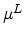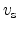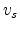Next: 4.3 Bulk Mobility of Up: 4. Mobility Modeling Previous: 4.1 Semiconductor Device Equations

Subsections

# 4.2 Modeling Approaches

The mobility behavior in a MOS transistor can be classified into: (i) low field bulk mobility (ii) surface/inversion layer mobility and (iii) high field mobility. In the low electric field regime, the charge carriers are almost in equilibrium with the lattice and the mobility has a characteristic low-field value that is commonly denoted by the symbol. The value of this mobility is dependent upon phonon and impurity scattering.

Modeling of carriers in the inversion layer poses additional complications. In the inversion layer, the carriers are subject to surface scattering, carrier-carrier scattering and quantum mechanical confinement. All these effects cause mobility degradation. The transverse electric field originating from the applied gate bias often serves as a parameter to indicate the strength of the inversion layer phenomena.

The high field behavior shows that the carrier mobility declines with electric field because the carriers that gain energy experience larger scattering rates. The mean drift velocity no longer increases linearly with increasing electric field, but rises more slowly. Eventually, the velocity saturates at a constant value. This saturation velocity is commonly denoted by the symbol. Impurity scattering is relatively insignificant for energetic carriers, and sois primarily a function of the lattice temperature.

The modeling approaches of physical parameters such as the mobility can be subdivided into three categories: [Mujtaba95]

1. Physically based: Mathematical expressions describing the physical effects are derived using first principle calculations. To capture the entire physical model into a closed form solution in which the parameter dependencies are obtained from fundamental calculations, considerable simplifying assumptions have to be made.

2. Semi-empirical: This approach arises because in practice it is seldom the case that the physically-based models conform to the experimental data. Therefore, to reconcile the model with experimental data, the coefficients appearing in the physically-based model are allowed to vary from their original values while certain dependencies resulting from the first principles calculation are retained.

3. Empirical: Empirically-based models are those in which all dependencies are allowed to vary. Compared to the other two approaches, this modeling approach generally tends to obscure the important physics thereby exhibiting a narrower range of validity. Empirical models are usually resorted to when the dependencies predicted by the first principles calculation do not allow a good fit between the experimental data and the corresponding semi-empirical model. These models are usually more efficient in comparison to the other two modeling approaches.

## 4.2.1 Monte Carlo Method

The Monte Carlo (MC) method is a widely used statistical numerical technique for simulating the behavior of various complex physical and mathematical systems. The method was first applied by Kurosawa [Kurosawa66] for solving the Boltzmann transport equation which governs the motion of electrons and holes in semiconductors. Within this technique a particle trajectory is constructed as a sequence of free flights and scattering events. The free flight times between collisions and the parameters of the scattering events are generated stochastically using probabilities of the microscopic processes. Thus the main advantage of this approach is the direct description of the microscopic particle dynamics. The numerical band structures of different semiconductors can be taken into account. The method was subsequently improved and applied to comprehensively study the electron transport in Si and Ge [Jacoboni83].

A distinct advantage of the Monte Carlo method is its ability to provide a solution of the BTE without compromising on the basic physical models for band structure and scattering processes. However, due to the statistical nature of the method, large simulation times are needed for performing full device simulations, in order to obtain meaningful average quantities of interest and to reduce the stochastic error.

In this work, the effect of strain on the electronic transport properties has been investigated using the Vienna Monte Carlo Simulator (VMC). It offers simulation algorithms for both bulk semiconductors and one-dimensional devices based on analytical band and full-band models. Additionally, a fast zero-field algorithm is included [Smirnov03]. VMC provides a mature set of scattering models including phonon scattering, ionized impurity scattering, alloy scattering, and impact ionization. Details concerning the scattering models implemented can be found in the documentation [IuE06].Next: 4.3 Bulk Mobility of Up: 4. Mobility Modeling Previous: 4.1 Semiconductor Device Equations

S. Dhar: Analytical Mobility Modeling for Strained Silicon-Based Devices